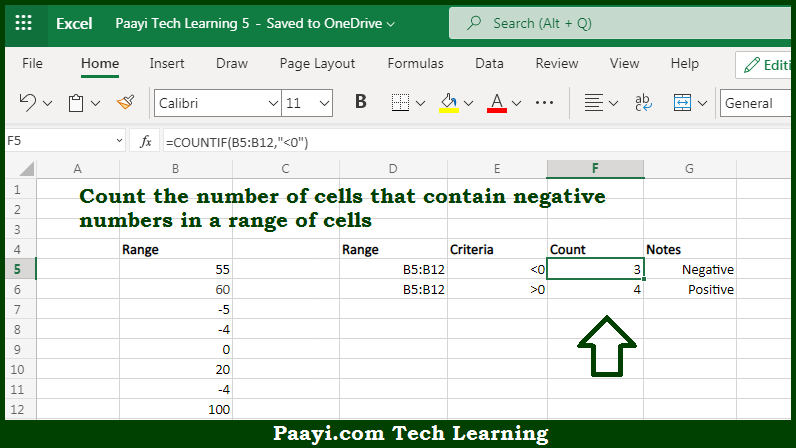# Learn How to Count Cells that Contain Negative Numbers in Microsoft Excel

Written by | 0 Comments | 533 Views

In this article, you will learn how to COUNT various things in Microsoft Excel using a single or combination of functions and its purpose in Microsoft Excel. You will also get to know how to count cells that contain negative numbers and see the generic

Count Cells that Contain Negative Numbers in Microsoft Excel

The main purpose of this formula is to count the number of cells that contain negative numbers in a range of cells. Here we will learn how to count cells having negative numbers in Microsoft Excel.  That implies, with the help of a formula based on the  COUNTIF function you can able to count the number of cells that contain negative numbers in a range of cells. The generic form of the formula provided below "rng" represents a range of cells that contain numbers. So, with the help of this formula, you can able to count the number of cells that contain negative numbers in a range of cells.

General Formula to COUNT Cells that Contain Negative Numbers

=COUNTIF(rng,"<0")

The Explanation for the COUNT Cells that Contain Negative NumbersSo we know that with the help of the given formula above you can able to count the number of cells that contain negative numbers in a range of cells. Here we will learn how to count cells having negative numbers in Microsoft Excel. As we know that, when you use the COUNTIF function you can able to counts the number of cells in a range that match the supplied criteria. In the example provided here, the criteria are supplied as "<0", the evaluation of that is "values less than zero". It should be noted that the total count of all cells in the range that meet these criteria is returned by the function. So, with the help of this formula, you can able to count cells that contain negative numbers.